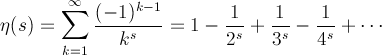Computes the Dirichlet eta function.

Namespace:  Meta.Numerics.Functions
Assembly:  Meta.Numerics (in Meta.Numerics.dll) Version: 4.1.4Syntax
```public static double DirichletEta(
double x
)```

#### Parameters

x
Type: SystemDouble
The argument, which must be non-negative.

#### Return Value

Type: Double
The value of η(s).Exceptions
ExceptionCondition
ArgumentOutOfRangeExceptionx is negative.Remarks

The Dirichlet eta function is the sum of the xth inverse power of the natural numbers, with alternating signs.Because these are just the terms of the Riemann zeta function (RiemannZeta(Double)) with alternating signs, it is also called the alternating zeta function.

It can be related to the Riemann ζ function.See Also